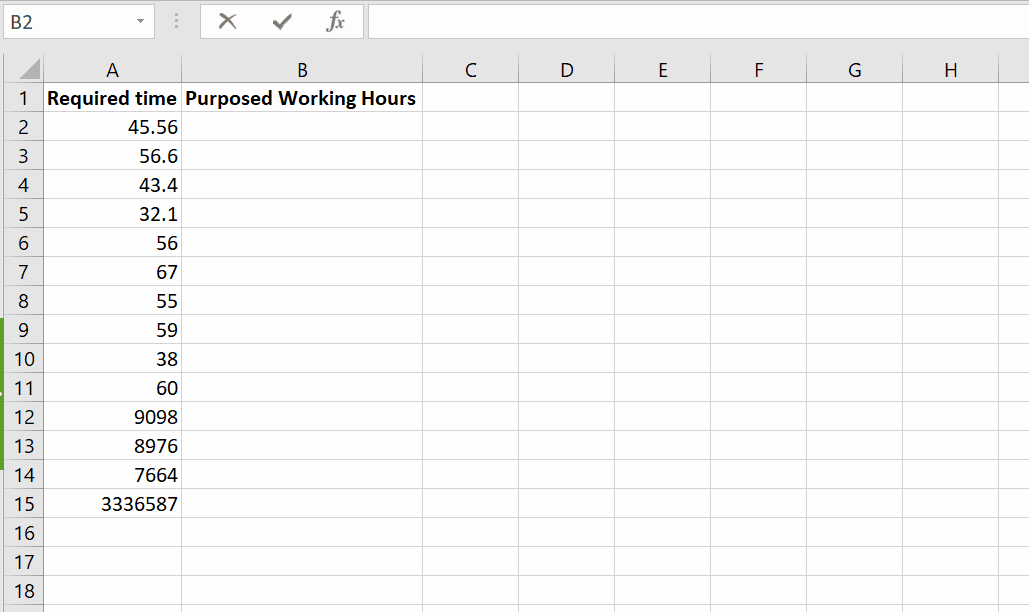# How to round to nearest 5 in Excel

In your spreadsheets while performing calculations you might need to round up a number to simplify calculations or avoid over insights. So, let’s see how we can round up a number to 5 in excel.Microsoft Excel has a lot of built-in functions for the ease of users. As Excel gives us the ability of data manipulation, likewise, there is another tremendous feature of Excel which is used to round up numbers to our desired nearest value.

There is an amazing feature of MROUNDUP for decimal data management.

### Step 1 – Write the formula– Select the cell you want to use for the expression and press F2 key on the keyboard or you can double click the cell as well. You can also write the formula in the formula bar once you selected the cell.

– Write the formula,

=MROUNDUP(number, multiple)

– The number will be the number to be rounded off and multiple will be the value to which the given numbers will be rounded up. Here we are using 5 to indicate that we want to round up to multiple of 5.

Hence, it rounded up the data range to the nearest multiple of 5.